# Introduction to R Graphics

Le Yan
HPC@LSU

## h2

### R Graphic Systems

• There are at least three plotting systems in R
• base
• lattice
• ggplot2
• Today we will touch on “base” briefly then focus on “ggplot2”

### Outline

• Base plot system
• ggplot2 plot system
• Basic concepts
• Geom and stat functions
• Title, axis labels and legends
• Themes
• Scale functions
• Coordination systems
• Faceting

### DataSets

• Datasets are from the packages `datasets`, `gcookbook` and`ggplot2“
• To learn more about those datasets, run `help(library='<name>')`
• For information on individual datasets, run `?<dataset>`
``````library(help='datasets')
``````
``````        Information on package 'datasets'

Description:

Package:       datasets
Version:       3.3.3
Priority:      base
Title:         The R Datasets Package
Author:        R Core Team and contributors worldwide
Maintainer:    R Core Team <R-core@r-project.org>
Description:   Base R datasets.
Built:         R 3.3.3; ; 2017-03-06 14:15:22 UTC; windows

Index:

AirPassengers           Monthly Airline Passenger Numbers 1949-1960
BJsales                 Sales Data with Leading Indicator
BOD                     Biochemical Oxygen Demand
CO2                     Carbon Dioxide Uptake in Grass Plants
ChickWeight             Weight versus age of chicks on different diets
DNase                   Elisa assay of DNase
EuStockMarkets          Daily Closing Prices of Major European Stock
...
``````

### First Plot in R

• We will use the “pressure” dataset for our first plot.

First, let's examine the data:

``````str(pressure)
``````
``````'data.frame':   19 obs. of  2 variables:
\$ temperature: num  0 20 40 60 80 100 120 140 160 180 ...
\$ pressure   : num  0.0002 0.0012 0.006 0.03 0.09 0.27 0.75 1.85 4.2 8.8 ...
``````
``````summary(pressure)
``````
``````  temperature     pressure
Min.   :  0   Min.   :  0.0002
1st Qu.: 90   1st Qu.:  0.1800
Median :180   Median :  8.8000
Mean   :180   Mean   :124.3367
3rd Qu.:270   3rd Qu.:126.5000
Max.   :360   Max.   :806.0000
``````
``````?pressure
``````
``````pressure {datasets} R Documentation
Vapor Pressure of Mercury as a Function of Temperature

Description

Data on the relation between temperature in degrees Celsius and vapor pressure of mercury in millimeters (of mercury).

Usage

pressure
Format

A data frame with 19 observations on 2 variables.

[, 1]    temperature     numeric     temperature (deg C)
[, 2]    pressure    numeric     pressure (mm)
Source

Weast, R. C., ed. (1973) Handbook of Chemistry and Physics. CRC Press.

References

McNeil, D. R. (1977) Interactive Data Analysis. New York: Wiley.
``````

### First Plot in R

We can use the `plot()` function in the base plot system to create a scatter plot:

``````# Simply specify the x and y variables.
plot(pressure\$temperature,pressure\$pressure)
``````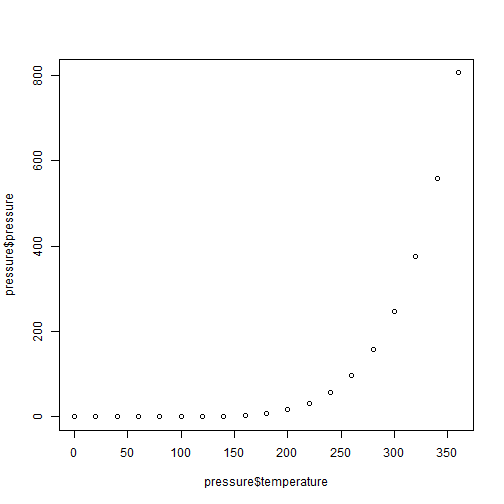Since there are only two variables, we can simply run:

``````plot(pressure)
``````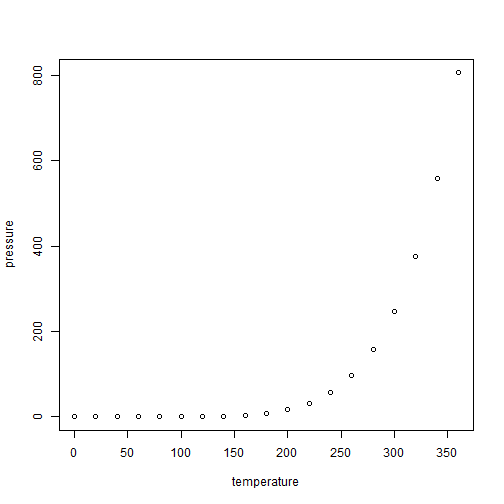### More Plot Types

• The `type` argument of `plot()` can be used to specify plot type

Line plot with “l”:

``````plot(pressure,type="l")
``````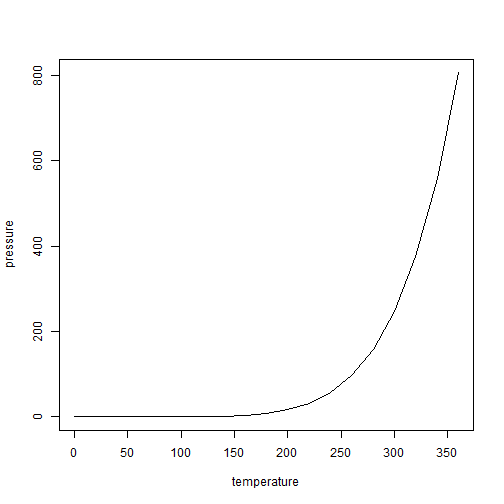Or dot and line with “b”:

``````plot(pressure,type="b")
``````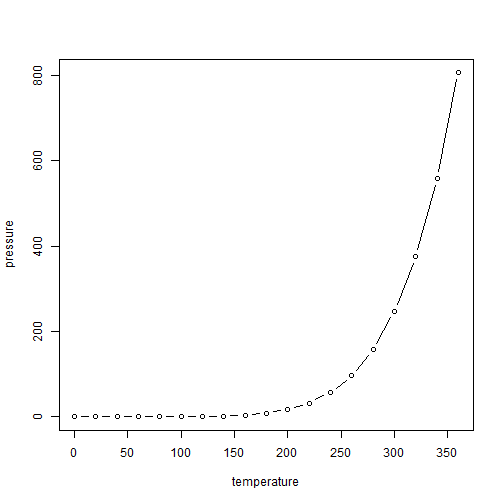There are a few functions that can be used to add more elements/layers to the plot

• Points
• Lines
• Texts
``````# Create the plot with title and axis labels.
plot(pressure,type="l",
main="Vapor Pressure of Mercury",
xlab="Temperature",
ylab="Vapor Pressure")

points(pressure,size=4,col='red')

text(150,700,"Source: Weast, R. C., ed. (1973) Handbook \n
of Chemistry and Physics. CRC Press.")
``````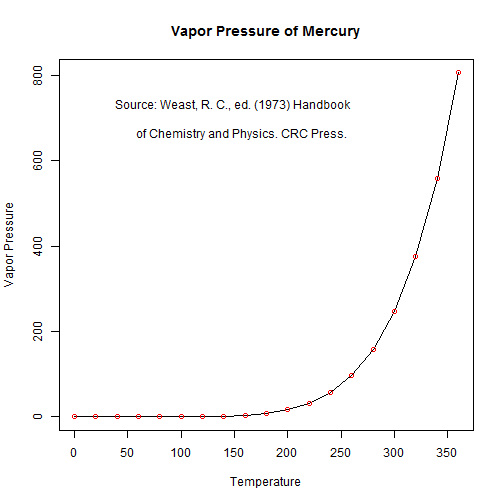### Boxplot

• Use `boxplot()` for boxplots

dataset:

``````str(mpg)
``````
``````Classes 'tbl_df', 'tbl' and 'data.frame':   234 obs. of  11 variables:
\$ manufacturer: chr  "audi" "audi" "audi" "audi" ...
\$ model       : chr  "a4" "a4" "a4" "a4" ...
\$ displ       : num  1.8 1.8 2 2 2.8 2.8 3.1 1.8 1.8 2 ...
\$ year        : int  1999 1999 2008 2008 1999 1999 2008 1999 1999 2008 ...
\$ cyl         : int  4 4 4 4 6 6 6 4 4 4 ...
\$ trans       : chr  "auto(l5)" "manual(m5)" "manual(m6)" "auto(av)" ...
\$ drv         : chr  "f" "f" "f" "f" ...
\$ cty         : int  18 21 20 21 16 18 18 18 16 20 ...
\$ hwy         : int  29 29 31 30 26 26 27 26 25 28 ...
\$ fl          : chr  "p" "p" "p" "p" ...
\$ class       : chr  "compact" "compact" "compact" "compact" ...
``````
``````summary(mpg)
``````
`````` manufacturer          model               displ            year
Length:234         Length:234         Min.   :1.600   Min.   :1999
Class :character   Class :character   1st Qu.:2.400   1st Qu.:1999
Mode  :character   Mode  :character   Median :3.300   Median :2004
Mean   :3.472   Mean   :2004
3rd Qu.:4.600   3rd Qu.:2008
Max.   :7.000   Max.   :2008
cyl           trans               drv                 cty
Min.   :4.000   Length:234         Length:234         Min.   : 9.00
1st Qu.:4.000   Class :character   Class :character   1st Qu.:14.00
Median :6.000   Mode  :character   Mode  :character   Median :17.00
Mean   :5.889                                         Mean   :16.86
3rd Qu.:8.000                                         3rd Qu.:19.00
Max.   :8.000                                         Max.   :35.00
hwy             fl               class
Min.   :12.00   Length:234         Length:234
1st Qu.:18.00   Class :character   Class :character
Median :24.00   Mode  :character   Mode  :character
Mean   :23.44
3rd Qu.:27.00
Max.   :44.00
``````
``````?mpg
``````
``````Fuel economy data from 1999 and 2008 for 38 popular models of car

Description

This dataset contains a subset of the fuel economy data that the EPA makes available on http://fueleconomy.gov. It contains only models which had a new release every year between 1999 and 2008 - this was used as a proxy for the popularity of the car.

Usage

mpg
Format

A data frame with 234 rows and 11 variables

manufacturer
model
model name

displ
engine displacement, in litres

year
year of manufacture

cyl
number of cylinders

trans
type of transmission

drv
f = front-wheel drive, r = rear wheel drive, 4 = 4wd

cty
city miles per gallon

hwy
highway miles per gallon

fl
fuel type

class
"type" of car
``````

### Boxplot

``````boxplot(hwy ~ cyl, data=mpg)

# Use the title function to add title and labels.
title("Highway Mileage per Gallon",
xlab = "Number of cylinders",
ylab = "Mileage (per gallon)")
``````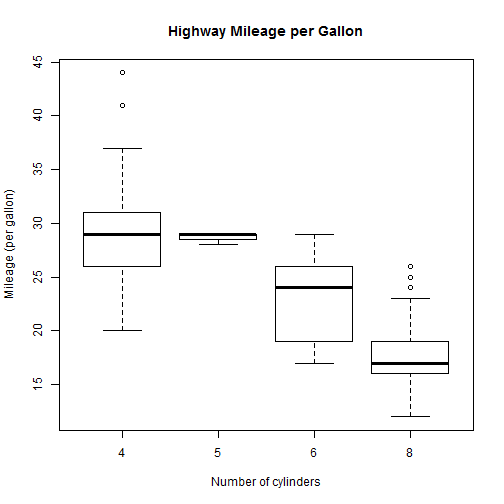### Histogram

• Function `hist()` can used to create histograms
``````hist(mpg\$hwy)
``````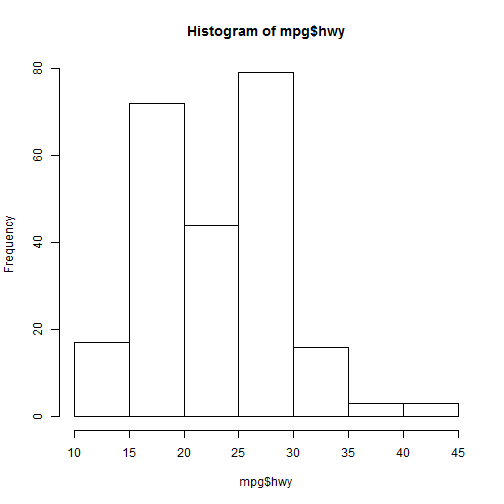``````hist(mpg\$hwy, breaks=c(5,15,25,30,50))
``````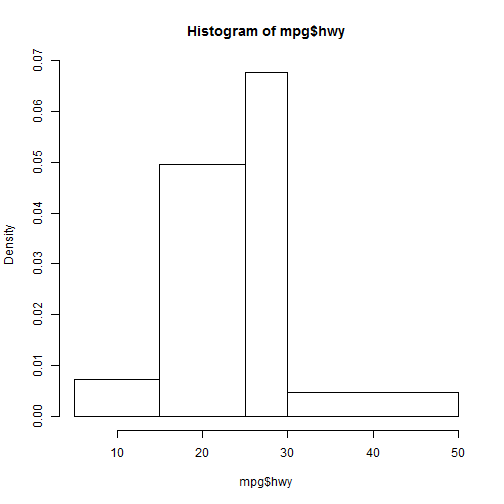### Curve

The `curve()` function draws a function over a specified range.

``````curve(cos,-3*pi, 3*pi)
title("Cosine Function")

# The abline() function adds one or more straight lines to the current plot
abline(h=c(-1,0,1),
col = 2, lty = 2, lwd = 1.5)
``````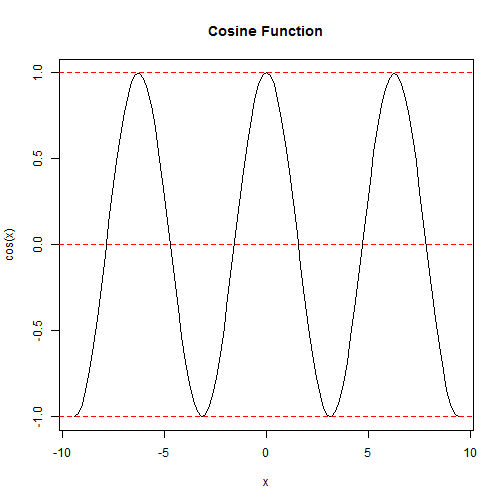### Panel Grid of Plots

• When datasets with multiple variables are passed to `plot()` without X and Y being specified, it will generate a panel grid of plots.
``````str(airquality)
``````
``````'data.frame':   153 obs. of  6 variables:
\$ Ozone  : int  41 36 12 18 NA 28 23 19 8 NA ...
\$ Solar.R: int  190 118 149 313 NA NA 299 99 19 194 ...
\$ Wind   : num  7.4 8 12.6 11.5 14.3 14.9 8.6 13.8 20.1 8.6 ...
\$ Temp   : int  67 72 74 62 56 66 65 59 61 69 ...
\$ Month  : int  5 5 5 5 5 5 5 5 5 5 ...
\$ Day    : int  1 2 3 4 5 6 7 8 9 10 ...
``````
``````plot(airquality)
``````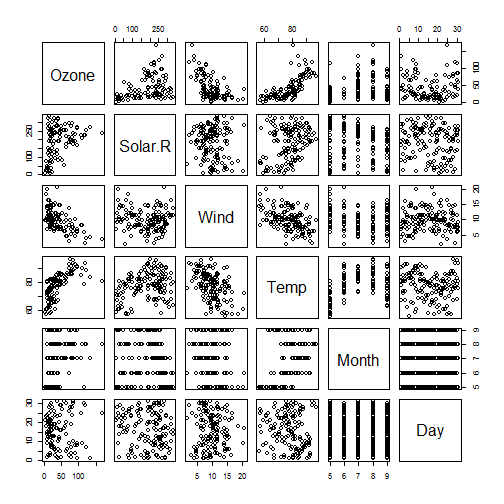### Saving Plots to Files

• Steps to save a plot to a file
• Open a device
• Create the plot
• Close the device
• Supported devices
• Vector: svg
• Bitmap: jpeg,tiff,png,bmp
• PDF: pdf
• Postcript: postcript

Example: saving to a PNG file

``````png("test.png",width=5*240,height=3*240)
plot(pressure, type="l")
points(pressure,col="red")
dev.off()
``````

Here is the saved graph: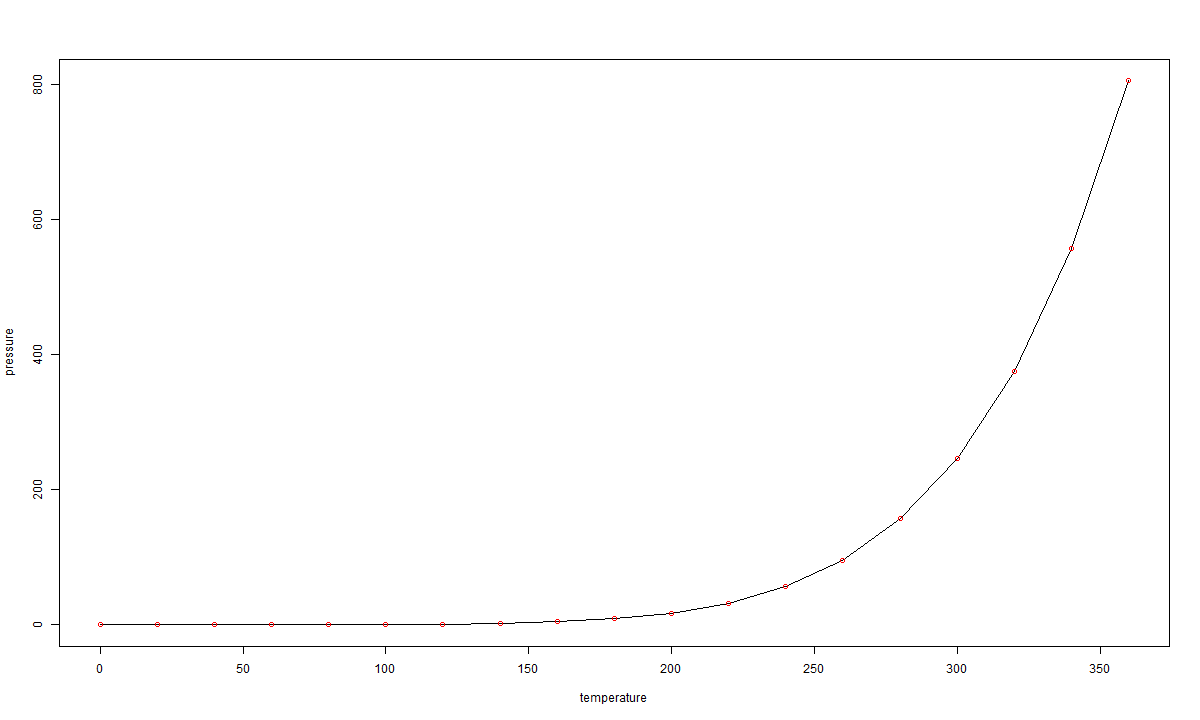### ggplot2 Package

• “gg” stands for grammar-of-graphics
• Any data graphics can be described by specifying
• A dataset
• Visual marks that represent data points
• A coordination system
• `ggplot2` package in R is an implementation of it
• Versatile
• Clear and consistent interface
• Beautiful output

### qplot Function

• The `qplot()` function from `ggplot2` package is similar to the `plot()` function in the base system.

Examine the data:

``````str(heightweight)
``````
``````'data.frame':   236 obs. of  5 variables:
\$ sex     : Factor w/ 2 levels "f","m": 1 1 1 1 1 1 1 1 1 1 ...
\$ ageYear : num  11.9 12.9 12.8 13.4 15.9 ...
\$ ageMonth: int  143 155 153 161 191 171 185 142 160 140 ...
\$ heightIn: num  56.3 62.3 63.3 59 62.5 62.5 59 56.5 62 53.8 ...
\$ weightLb: num  85 105 108 92 112 ...
``````
``````summary(heightweight)
``````
`````` sex        ageYear         ageMonth        heightIn        weightLb
f:111   Min.   :11.58   Min.   :139.0   Min.   :50.50   Min.   : 50.5
m:125   1st Qu.:12.33   1st Qu.:148.0   1st Qu.:58.73   1st Qu.: 85.0
Median :13.58   Median :163.0   Median :61.50   Median :100.5
Mean   :13.67   Mean   :164.1   Mean   :61.34   Mean   :101.0
3rd Qu.:14.83   3rd Qu.:178.0   3rd Qu.:64.30   3rd Qu.:112.0
Max.   :17.50   Max.   :210.0   Max.   :72.00   Max.   :171.5
``````
``````?heightweight
``````
``````heightweight {gcookbook}    R Documentation
Height and weight of schoolchildren

Description

Height and weight of schoolchildren

Variables

sex

ageYear: Age in years.

ageMonth: Age in months.

heightIn: Height in inches.

weightLb: Weight in pounds.

Source

Lewis, T., & Taylor, L.R. (1967), Introduction to Experimental Ecology, Academic Press.
``````

### Scatterplot with ```qplot``` Function

• Data represented by points
``````qplot(weightLb, heightIn, data=heightweight, geom="point")
``````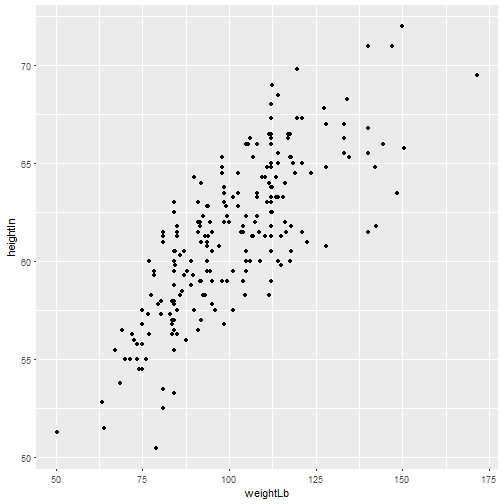• Data represented by labels
``````qplot(weightLb, heightIn, data=heightweight, geom ="text", label=ageYear)
``````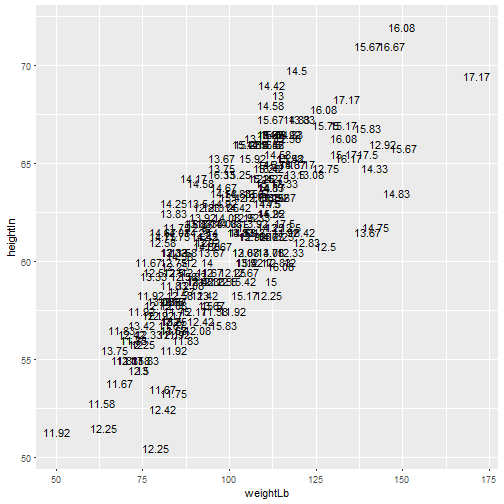### A Fancier Plot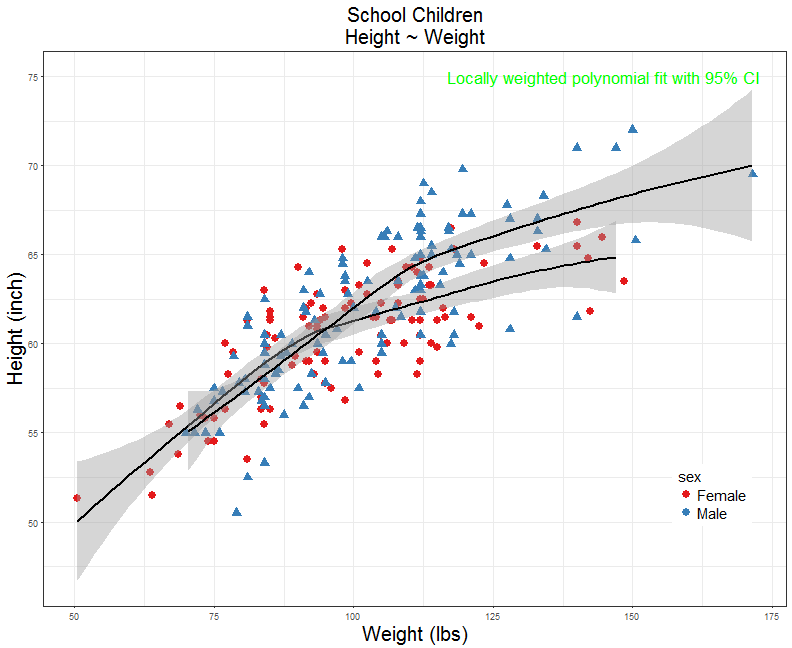### A Fancier Plot

This is what is under the hood:

``````ggplot(heightweight, aes(x=weightLb, y=heightIn, color=sex, shape=sex)) +
geom_point(size=3.5) +
ggtitle("School Children\nHeight ~ Weight") +
labs(y="Height (inch)", x="Weight (lbs)") +
stat_smooth(method=loess, se=T, color="black", fullrange=T) +
annotate("text",x=145,y=75,label="Locally weighted polynomial fit with 95% CI",color="Green",size=6) +
scale_color_brewer(palette = "Set1", labels=c("Female", "Male")) +
guides(shape=F) +
theme_bw() +
theme(plot.title = element_text(size=20, hjust=0.5),
legend.position = c(0.9,0.2),
axis.title.x = element_text(size=20), axis.title.y = element_text(size=20),
legend.title = element_text(size=15),legend.text = element_text(size=15))
``````

Don't Panic!!!

### Basic Concepts of ggplot2

Grammar of Graphics components:

• Data: Use the `ggplot` function to indicate what